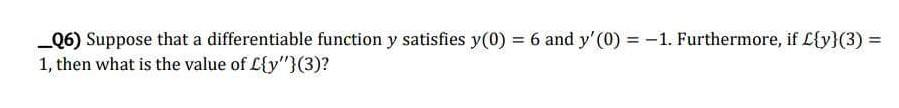Home / Expert Answers / Calculus / nbsp-6-suppose-that-a-differentiable-function-y-satisfies-y-0-6-and-y-pri-pa289

# (Solved):   6) Suppose that a differentiable function $$y$$ satisfies $$y(0)=6$$ and $$y^{\pri ...—6) Suppose that a differentiable function \( y$$ satisfies $$y(0)=6$$ and $$y^{\prime}(0)=-1$$. Furthermore, if $$\mathcal{L}\{y\}(3)=$$ 1 , then what is the value of $$\mathcal{L}\left\{y^{\prime \prime}\right\}(3)$$ ?

We have an Answer from Expert